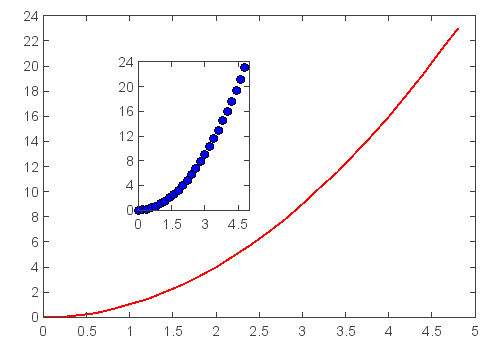# Plot tutorial¶

MeteoInfoLab has a collection of command style functions that make MeteoInfoLab work like MATLAB. Each plot function makes some change to a figure: e.g., create a figure, create a plotting area in a figure, plot some lines in a plotting area, decorate the plot with lables, etc…. MeteoInfoLab is stateful, in that it keeps track of the current figure and plotting area, and the plotting functions are directed to the current axes.

```plot([1,2,3,4])
ylabel('some numbers')
```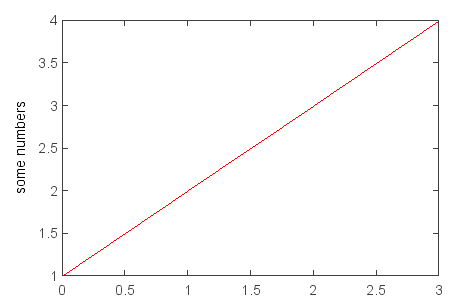You may be wondering why the x-axis ranges from 0-3 and y-axis from 1-4. If you provide a single list or array to the plot() command, MeteoInfoLab assumes it is a sequence of y values, and automatically generates the x vaues for you. Since python ranges start with 0, the default x vector has the same length as y but starts with 0. Hence the x data are [0,1,2,3].

plot() is a versatile command, and will take an arbitrary number of arguments. For example, to plot x versus y, you can issue the command:

```plot([1,2,3,4], [1,4,9,16])
```

For every x, y pair of arguments, there is an optional third argument which is the format string that indicates the color and line type of the plot. The letters and symbols of the format string are from MATLAB, and you concatenate a color string with a line style string. The default format string is ‘r-’, which is a solid red line. For example, to plot the above with red circles, you would issue

```plot([1,2,3,4], [1,4,9,16], 'ro')
axis([0, 6, 0, 20])
```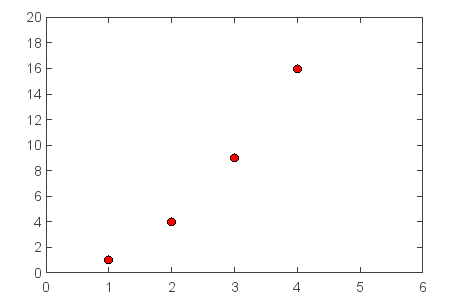See the plot() documentation for a complete list of line styles and format strings. The axis() command in the example above takes a list of `[xmin, xmax, ymin, ymax]` and specifies the viewport of the axes.

Arrays are useful for numerical processing. The example below illustrates a plotting several lines with different format styles in one command using arrays.

```# evenly sampled time at 200ms intervals
t = arange(0., 5., 0.2)

# red dashes, blue squares and green triangles
plot(t, t, 'r--', t, t**2, 'bs', t, t**3, 'g^')
```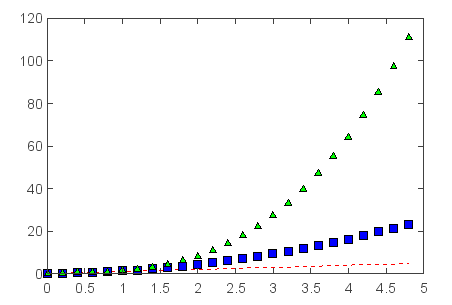## Controlling line properties¶

Lines have many attributes that you can set: linewidth, style, etc; The way to set line properties

• Use keyword args:

```plot(x, y, linewidth=2.0)
```

## Working with multiple axes¶

MATLAB, and MeteoInfoLab, have the concept of the current figure and the current axes. All plotting commands apply to the current axes. Normally, you don’t have to worry about this, because it is all taken care of the behind the scenes. Below is a script to create two subplots.

```def f(t):
return exp(-t) * cos(2*pi*t)

t1 = arange(0.0, 5.0, 0.1)
t2 = arange(0.0, 5.0, 0.02)

subplot(2,1,1)
plot(t1, f(t1), 'bo', t2, f(t2), 'k')

subplot(2,1,2)
plot(t2, cos(2*pi*t2), 'r--')
```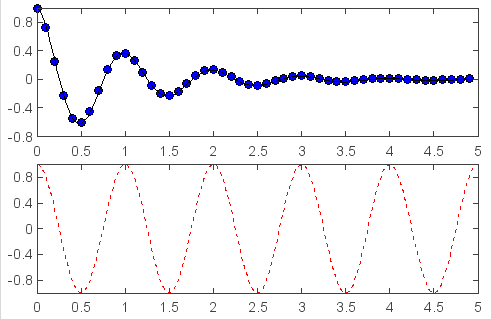The subplot() command specifies `numrows, numcols, fignum` ranges from 1 to `numrows*numcols`. You can create an arbitrary number of subplots and axes. If you want to place an axes manually, i.e., not on a rectangular grid, use the axes() command, which allows you to specify the location as `axes(position=[left, bottom, width, height])` where all values are in fractional (0 to 1) coordinates.

```x = arange(0., 5., 0.2)
y = x**2
plot(x, y, label='Series_1', linewidth=2.0)
axes(position=[0.3,0.4,0.2,0.4])
plot(x, y, 'bo')
```